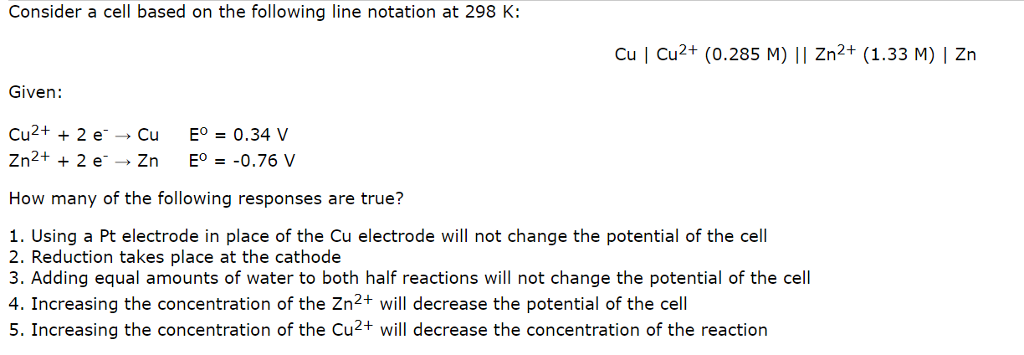# Question & Answer: Consider a cell based on the following line notation at 298 K: Cu | Cu^2+ (0.285 M) || Zn^2+…..Consider a cell based on the following line notation at 298 K: Cu | Cu^2+ (0.285 M) || Zn^2+ (1.33 M) | Zn Given: Cu^2+ + 2 e^- rightarrow Cu E^0 = 0.34 V Zn^2+ + 2 e^- rightarrow Zn E^0 = -0.76 V How many of the following responses are true? Using a Pt electrode in place of the Cu electrode will not change the potential of the cell Reduction takes place at the cathode Adding equal amounts of water to both half reactions will not change the potential of the cell Increasing the concentration of the Zn^2+ will decrease the potential of the cell Increasing the concentration of the Cu^2+ will decrease the concentration of the reaction

1. This is true. The cell potential is basically the difference of potential of two electrode reactions. As the reactions at the electrodes are same, the cell potential will not change.

Don't use plagiarized sources. Get Your Custom Essay on
Question & Answer: Consider a cell based on the following line notation at 298 K: Cu | Cu^2+ (0.285 M) || Zn^2+…..
GET AN ESSAY WRITTEN FOR YOU FROM AS LOW AS \$13/PAGE

2. This statement is true.

3. This is true. As adding equal amount of water to both half reactions will decrease the concentration of both Zn+2 and Cu+2 to same extent and the net effect is 0. So, there will be no change in cell potential.

4. The cell reaction is:

Cu+2 + Zn = Zn+2 + Cu

Here Zn+2 is produced and if the concentration of Zn+2 increases, ratio of concentration of Zn+2 and Cu+2 increases. And according to Nernst equation: cell potential decreases.

So, the statement is true.

5. This statement is not true. As concentration of Cu+2 increases, [Zn+2] also increases as more Zn+2 will be produced in the reaction. So reaction concentration increases.